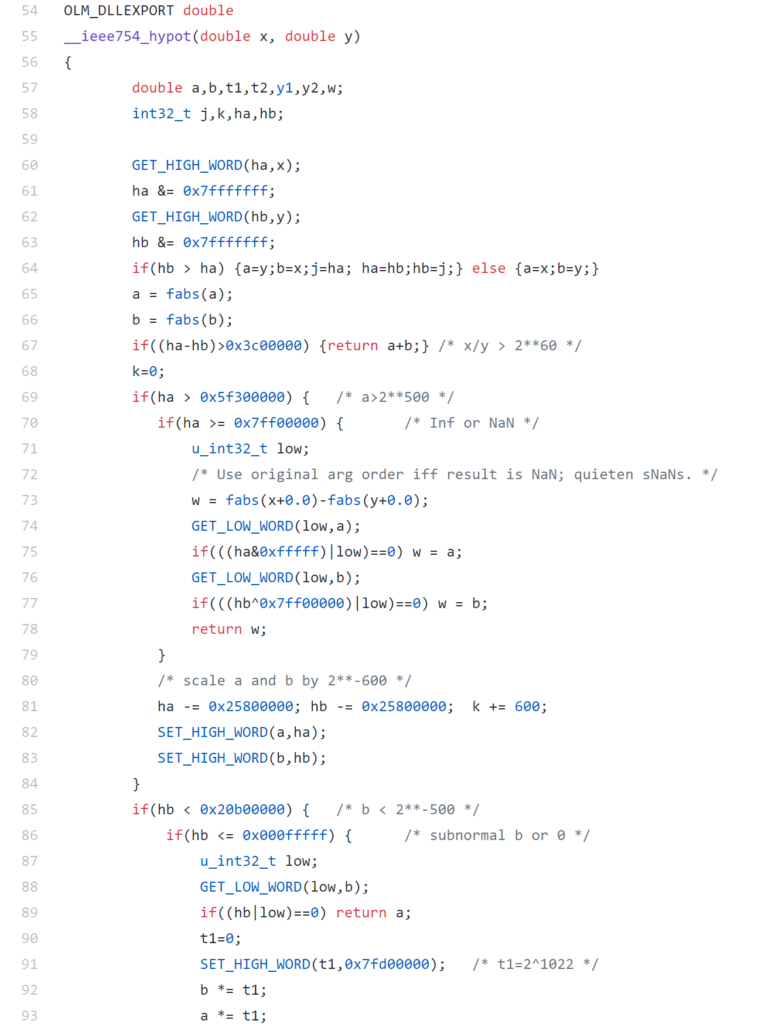## Hypot – A story of a ‘simple’ function

January 6th, 2020

My stepchildren are pretty good at mathematics for their age and have recently learned about Pythagora’s theorem

$c=\sqrt{a^2+b^2}$

The fact that they have learned about this so early in their mathematical lives is testament to its importance. Pythagoras is everywhere in computational science and it may well be the case that you’ll need to compute the hypotenuse to a triangle some day.

Fortunately for you, this important computation is implemented in every computational environment I can think of!
It’s almost always called hypot so it will be easy to find.
Here it is in action using Python’s numpy module

import numpy as np
a = 3
b = 4
np.hypot(3,4)

5


When I’m out and about giving talks and tutorials about Research Software Engineering, High Performance Computing and so on, I often get the chance to mention the hypot function and it turns out that fewer people know about this routine than you might expect.

### Trivial Calculation? Do it Yourself!

Such a trivial calculation, so easy to code up yourself! Here’s a one-line implementation

def mike_hypot(a,b):
return(np.sqrt(a*a+b*b))


In use it looks fine

mike_hypot(3,4)

5.0


### Overflow and Underflow

I could probably work for quite some time before I found that my implementation was flawed in several places. Here’s one

mike_hypot(1e154,1e154)

inf


You would, of course, expect the result to be large but not infinity. Numpy doesn’t have this problem

np.hypot(1e154,1e154)

1.414213562373095e+154


My function also doesn’t do well when things are small.

a = mike_hypot(1e-200,1e-200)

0.0


but again, the more carefully implemented hypot function in numpy does fine.

np.hypot(1e-200,1e-200)

1.414213562373095e-200


### Standards Compliance

Next up — standards compliance. It turns out that there is a an official standard for how hypot implementations should behave in certain edge cases. The IEEE-754 standard for floating point arithmetic has something to say about how any implementation of hypot handles NaNs (Not a Number) and inf (Infinity).

It states that any implementation of hypot should behave as follows (Here’s a human readable summary https://www.agner.org/optimize/nan_propagation.pdf)

hypot(nan,inf) = hypot(inf,nan) = inf


numpy behaves well!

np.hypot(np.nan,np.inf)

inf

np.hypot(np.inf,np.nan)

inf


My implementation does not

mike_hypot(np.inf,np.nan)

nan


So in summary, my implementation is

• Wrong for very large numbers
• Wrong for very small numbers
• Not standards compliant

That’s a lot of mistakes for one line of code! Of course, we can do better with a small number of extra lines of code as John D Cook demonstrates in the blog post What’s so hard about finding a hypotenuse?

### Hypot implementations in production

Production versions of the hypot function, however, are much more complex than you might imagine. The source code for the implementation used in openlibm (used by Julia for example) was 132 lines long last time I checked. Here’s a screenshot of part of the implementation I saw for prosterity. At the time of writing the code is at https://github.com/JuliaMath/openlibm/blob/master/src/e_hypot.cThat’s what bullet-proof, bug checked, has been compiled on every platform you can imagine and survived code looks like.

There’s more!

### Active Research

When I learned how complex production versions of hypot could be, I shouted out about it on twitter and learned that the story of hypot was far from over!

The implementation of the hypot function is still a matter of active research! See the paper here https://arxiv.org/abs/1904.09481### Is Your Research Software Correct?

Given that such a ‘simple’ computation is so complicated to implement well, consider your own code and ask Is Your Research Software Correct?.